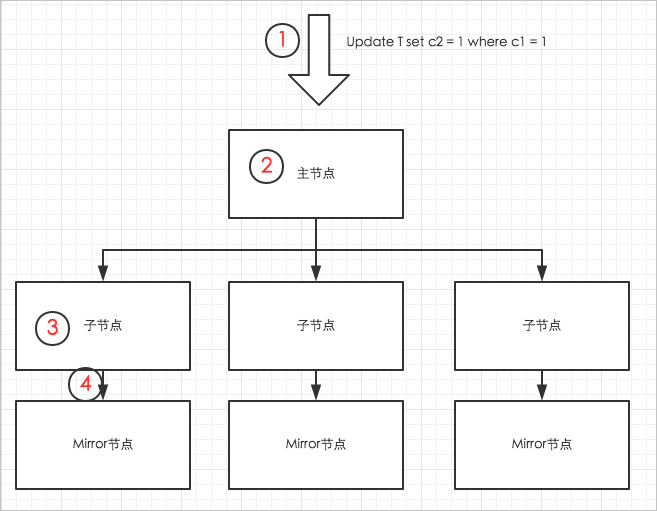## 简单更新过程1. 用户发送Update的SQL请求至主节点。
2. 主节点发起分布式事务，对被Update的表加锁（AnalyticDB for PostgreSQL不允许并行Update同一张表），然后把更新请求分发到对应的子节点。
3. 子节点通过索引扫描，定位到要更新的数据，并更新数据。对于列存表，更新逻辑就是删除旧的数据行，并在表的尾端写入新的数据行。列存表中被更新的数据页面会写入内存缓存区，对应的表文件长度的变化（因为尾端写入了数据，所以数据表对应的文件长度增大了）会写入日志（xlog文件）。
4. 在Update命令结束前，内存中被更新的数据页面和xlog日志，都要同步到Mirror节点。同步完成后，主节点结束分布式事务，返回用户执行成功的消息。

• 把更新放到一个SQL语句，减少语句解析、节点通信、数据同步等开销。
• 把更新放到一个事务，避免不必要的事务开销。

## 批量Update

1.准备目标表

``````create table target_table(c1 int, c2 int, primary key (c1));
insert into target_table select generate_series(1, 10000000);``````

2.准备中间表

``````create table source_table(c1 int, c2 int);
insert into source_table select generate_series(1, 100), generate_series(1,100);``````

3.批量更新

source_table数据准备好后，执行如下`update set … from … where ..`语句，即可实现批量的Update。

``````set optimizer=on;
update target_table set c2 = source_table.c2 from source_table where target_table.c1= source_table.c1;``````

``````=> explain update target_table set c2 = source_table.c2 from source_table where target_table.c1= source_table.c1;
QUERY PLAN
-----------------------------------------------------------------------------------------------------------------------------
Update  (cost=0.00..586.10 rows=25 width=1)
->  Result  (cost=0.00..581.02 rows=50 width=26)
->  Redistribute Motion 4:4  (slice1; segments: 4)  (cost=0.00..581.02 rows=50 width=22)
Hash Key: public.target_table.c1
->  Assert  (cost=0.00..581.01 rows=50 width=22)
Assert Cond: NOT public.target_table.c1 IS NULL
->  Split  (cost=0.00..581.01 rows=50 width=22)
->  Nested Loop  (cost=0.00..581.01 rows=25 width=18)
Join Filter: true
->  Table Scan on source_table  (cost=0.00..431.00 rows=25 width=8)
->  Index Scan using target_table_pkey on target_table  (cost=0.00..150.01 rows=1 width=14)
Index Cond: public.target_table.c1 = source_table.c1``````

``````postgres=> insert into source_table select generate_series(1, 1000), generate_series(1,1000);
INSERT 0 1000
postgres=> analyze source_table;
ANALYZE
postgres=> explain update target_table set c2 = source_table.c2 from source_table where target_table.c1= source_table.c1;
QUERY PLAN
------------------------------------------------------------------------------------------------------
Update  (cost=0.00..1485.82 rows=275 width=1)
->  Result  (cost=0.00..1429.96 rows=550 width=26)
->  Assert  (cost=0.00..1429.94 rows=550 width=22)
Assert Cond: NOT public.target_table.c1 IS NULL
->  Split  (cost=0.00..1429.93 rows=550 width=22)
->  Hash Join  (cost=0.00..1429.92 rows=275 width=18)
Hash Cond: public.target_table.c1 = source_table.c1
->  Table Scan on target_table  (cost=0.00..477.76 rows=2500659 width=14)
->  Hash  (cost=431.01..431.01 rows=275 width=8)
->  Table Scan on source_table  (cost=0.00..431.01 rows=275 width=8)``````

## 批量Delete

``delete from target_table using source_table where target_table.c1 = source_table.c1;``

``````explain delete from target_table using source_table where target_table.c1 = source_table.c1;
QUERY PLAN
-----------------------------------------------------------------------------------------------------
Delete (slice0; segments: 4)  (rows=50 width=10)
->  Nested Loop  (cost=0.00..41124.40 rows=50 width=10)
->  Seq Scan on source_table  (cost=0.00..6.00 rows=50 width=4)
->  Index Scan using target_table_pkey on target_table  (cost=0.00..205.58 rows=1 width=14)
Index Cond: target_table.c1 = source_table.c1``````

## 利用Delete + Insert做数据合并

• 如果预先知道待合入的数据，在目标表中都已经有对应的数据行，即可通过Update语句实现数据合入。
• 但多数情况下，待合入的数据中，一部分是在目标表中已存在记录的数据，还有一部分是新增的，目标表中没有对应记录。这种情况下，需要使用一次批量Delete+一次批量Insert。代码示例如下。
``````set optimizer=on;
delete from target_table using source_table where target_table.c1 = source_table.c1;
insert into target_table select * from source_table;``````

## 利用Values()表达式做实时更新

``````update target_table set c2 = t.c2 from (values(1,1),(2,2),(3,3),…(2000,2000)) as t(c1,c2) where target_table.c1=t.c1
delete from target_table using (values(1,1),(2,2),(3,3),…(2000,2000)) as t(c1,c2) where target_table.c1 = t.c1``````